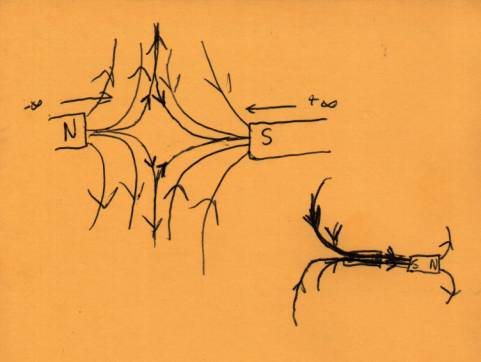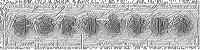# Common Field Theory (unfinished)

November 12, 2001

Version 1.0

In Common Field Theory the fundamental forces arise from the difference of High Field Pressure to Low Field Pressure Regions.  Such behavior is exhibited in a multitude of Fields: magnetic, electric, gravitational, and even macroscopic fields such as wind and fluid flows.

To see this principle more clearly we concentrate on the magnetic field.  First by concensus, magnetic field lines originate from the north pole of a given magnet and heads towards the south pole of a magnet.  Such a property is what causes a monopole magnet to ellude us for such a long time.  Without both the north and south poles, there are no field lines, and as such, no magnetic force.  From numerous experiements by past scientists, we can safely assume that like poles repel while different poles attract.

In order to see the effects of the fields from two opposite poles, we start the magnetic poles from a distance of effectively infinity.  None of the fields interact, so we observe no force.  Assume our North pole is at –infinity pointing towards the positive, and our south pole is at +infinity pointing towards the negative.  We will ignore the far end of each magnet for now.  As we bring the north and south pole together, we start to see fields overlapping.We notice that the field from the North pole and the field from the South pole are equal and opposite at the perpendicular to the motion and orientation of the magnets.  Bn = +b and Bs = -b.  So the sum of the fields that cross yield 0 Guass. This is true for all the perpendicular components of the magnetic fields in this region of overlapping fields.

On the other side of the magnet, we see that the perpendicular components due to both magnets are equal in direction, but the magnitude differs due to the distance.  But in any case, we observe that the sum of the fields is >Bn at the outer poles.  Thus this differential where the inner poles experience 0 perpendicular fields and the outer poles experience >Bn perpendicular fields causes High field pressure on the outside, and low field pressure on the inner poles.  The pressure difference creates a resultant force that brings the two magnets together.

Once we bring the two magnets together, we can see that on the inner poles, the remaining magnetic fields are all parallel to the two pole orientations, and from the north inner pole to the south inner pole.  This causes the two small magnets to exhibit field behavior of one larger magnet.

This is the principle of fundamental field behavior (FFB).  One might bring up electrons and claim that this is a counter example to the fundamental field behavior (FFB).  Since we see electrons generating fields toward the electron.  When we bring two electrons together, the two arrows are in opposite directions so they have low pressure in the region between the two electrons.  One would claim an attractive force based on FFB, but electrons repel each other.

The logic is sound but the assumption is wrong, but we must remember field pressure can only occur in a parallel orientation.  The two electrons have fields into the electron.  Draw a line between the two electrons and we can see that other than the field that is parallel to that line, all the other field lines have a parallel and a perpendicular component.  The parallel lines do cancel out, but the perpendicular lines reinforce each other resulting in a high-pressure region of field between the two electrons.  One can also see that the high pressure on the outer side will be less because of it’s distance to the furthest electron, therefore electrons would exhibit a repulsive force.

In a dipole electric scenario, the analysis is very similar to the magnetic dipole situation, therefore is left as an exercise to the reader.

This leads to quite an interesting situation with gravity field lines. From convention we assume that gravity fields points towards the source.  And we have yet to find a natural source of anti-gravity where field lines point away from the source.  If this is so, then how does two particle with gravity field lines pointing towards the source attract each other?

I propose that this mode of thought is faulty, and hence the current limitation in gravitational technology.  If two particles are gravitationally attracted to each other then based on FFB there must be high gravitational field pressure on outside of the two particles, and low gravitational field pressure on the insides.

Another fact arises which causes confusion in FFB, simply put, all particles are gravitationally attracted to other particles.  If a certain particle has "positive" gravity, then all other particles must have "negative" gravity.  But that contradicts the foundation, since now we have negative particles repelling other negative particles gravitationally.